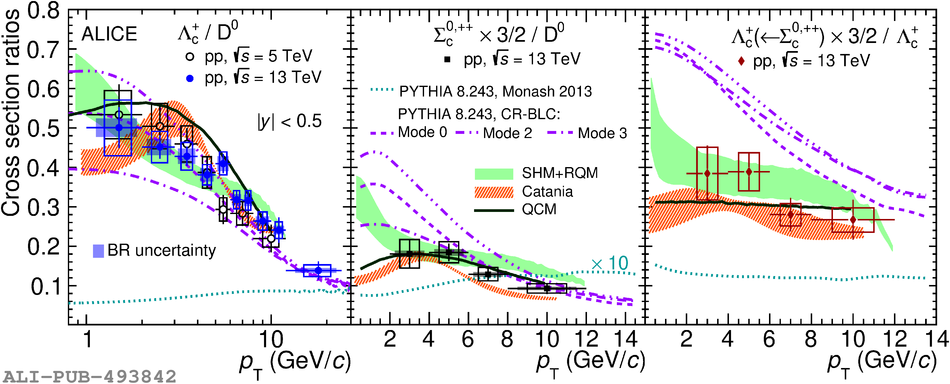# Figure 2

 Prompt-charm-hadron cross-section ratios: $\Lambdac/\Dzero$ (left), $\SigmacZeroPlusPlusPlus/\Dzero$ (middle), and $\LambdacFromScZeroPlusPlusPlus/\Lambdac$ (right), in pp collisions at $\sqrt{s}=13$\,\TeV, compared with model expectations~ and (left) with data from pp collisions at $\sqrt{s}=5.02$\,\TeV~. The horizontal lines reflect the width of the $\pt$ intervals. The PYTHIA Monash 2013 curve is scaled by a factor of 10 in the middle panel.